Anzeige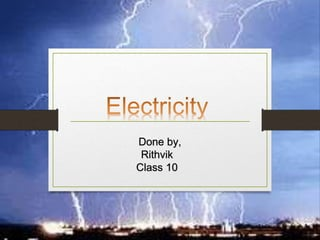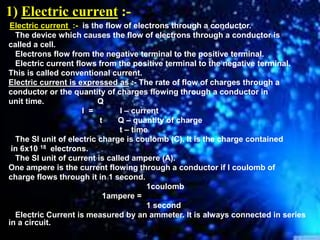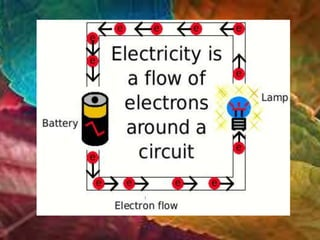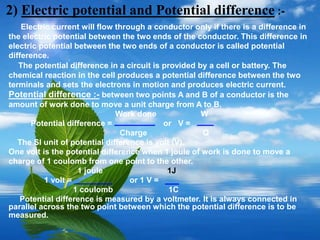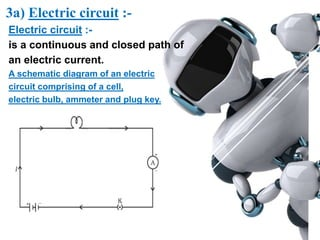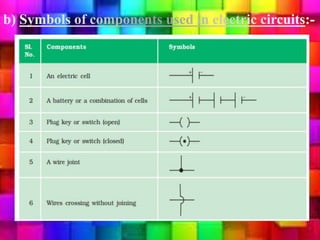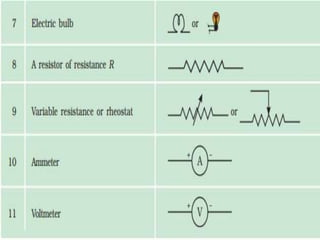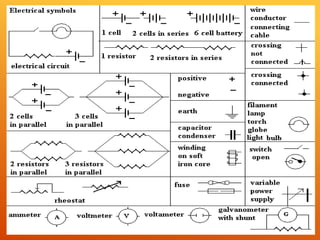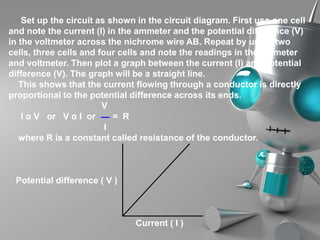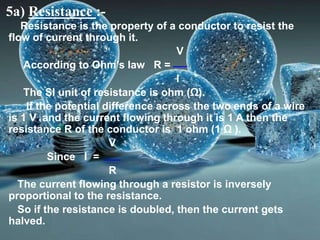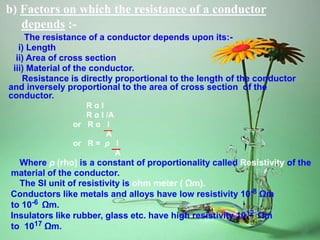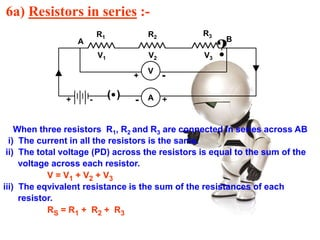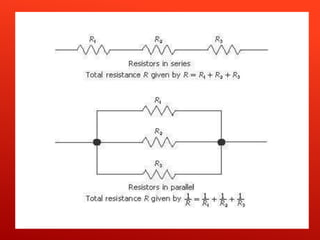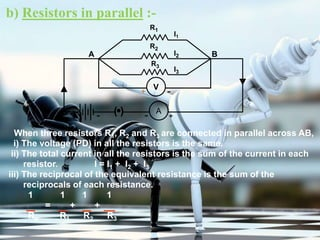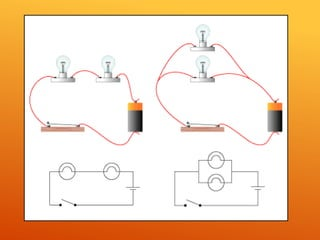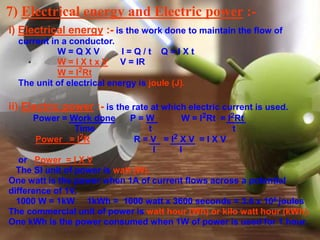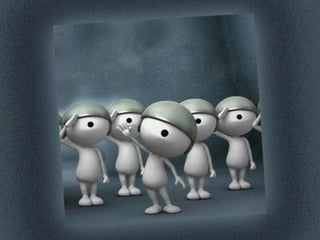1 von 20
Anzeige

### Chapter 12 Electricity class 10 ncert

1. Done by, Rithvik Class 10
2. 1) Electric current :- Electric current :- is the flow of electrons through a conductor. The device which causes the flow of electrons through a conductor is called a cell. Electrons flow from the negative terminal to the positive terminal. Electric current flows from the positive terminal to the negative terminal. This is called conventional current. Electric current is expressed as :- The rate of flow of charges through a conductor or the quantity of charges flowing through a conductor in unit time. Q I = I – current t Q – quantity of charge t – time The SI unit of electric charge is coulomb (C). It is the charge contained in 6x10 18 electrons. The SI unit of current is called ampere (A). One ampere is the current flowing through a conductor if I coulomb of charge flows through it in 1 second. 1coulomb 1ampere = 1 second Electric Current is measured by an ammeter. It is always connected in series in a circuit.
3. 2) Electric potential and Potential difference :- Electric current will flow through a conductor only if there is a difference in the electric potential between the two ends of the conductor. This difference in electric potential between the two ends of a conductor is called potential difference. The potential difference in a circuit is provided by a cell or battery. The chemical reaction in the cell produces a potential difference between the two terminals and sets the electrons in motion and produces electric current. Potential difference :- between two points A and B of a conductor is the amount of work done to move a unit charge from A to B. Work done W Potential difference = or V = Charge Q The SI unit of potential difference is volt (V). One volt is the potential difference when 1 joule of work is done to move a charge of 1 coulomb from one point to the other. 1 joule 1J 1 volt = or 1 V = 1 coulomb 1C Potential difference is measured by a voltmeter. It is always connected in parallel across the two point between which the potential difference is to be measured.
4. 3a) Electric circuit :- Electric circuit :- is a continuous and closed path of an electric current. A schematic diagram of an electric circuit comprising of a cell, electric bulb, ammeter and plug key.
5. 4) Ohm’s law :- Ohms law is a relationship between the potential difference across a conductor and the current flowing through it. Ohm’s law states that:- ‘The current flowing through a conductor is directly proportional to the potential difference between its ends provided its temperature remains constant.’ V V I α V or V α I or ---- = constant or ---- = R I I Where R is a constant called resistance for a given metallic wire at a given temperature. -
6. Set up the circuit as shown in the circuit diagram. First use one cell and note the current (I) in the ammeter and the potential difference (V) in the voltmeter across the nichrome wire AB. Repeat by using two cells, three cells and four cells and note the readings in the ammeter and voltmeter. Then plot a graph between the current (I) and potential difference (V). The graph will be a straight line. This shows that the current flowing through a conductor is directly proportional to the potential difference across its ends. V I α V or V α I or = R I where R is a constant called resistance of the conductor. Potential difference ( V ) Current ( I )
7. 5a) Resistance :- Resistance is the property of a conductor to resist the flow of current through it. V According to Ohm’s law R = I The SI unit of resistance is ohm (Ω). If the potential difference across the two ends of a wire is 1 V and the current flowing through it is 1 A then the resistance R of the conductor is 1 ohm (1 Ω ). V Since I = R The current flowing through a resistor is inversely proportional to the resistance. So if the resistance is doubled, then the current gets halved.
8. b) Factors on which the resistance of a conductor depends :- The resistance of a conductor depends upon its:- i) Length ii) Area of cross section iii) Material of the conductor. Resistance is directly proportional to the length of the conductor and inversely proportional to the area of cross section of the conductor. R α l R α I /A or R α l A or R = ρ l A Where ρ (rho) is a constant of proportionality called Resistivity of the material of the conductor. The SI unit of resistivity is ohm meter ( Ωm). Conductors like metals and alloys have low resistivity 10-8 Ωm to 10-6 Ωm. Insulators like rubber, glass etc. have high resistivity 1012 Ωm to 1017 Ωm.
9. 6a) Resistors in series :- When three resistors R1, R2 and R3 are connected in series across AB i) The current in all the resistors is the same. ii) The total voltage (PD) across the resistors is equal to the sum of the voltage across each resistor. V = V1 + V2 + V3 iii) The eqvivalent resistance is the sum of the resistances of each resistor. RS = R1 + R2 + R3 ( ) A R1 R2 R3 + +-- V1 V2 V3 A B V + -
10. b) Resistors in parallel :- When three resistors R1, R2 and R3 are connected in parallel across AB, i) The voltage (PD) in all the resistors is the same. ii) The total current in all the resistors is the sum of the current in each resistor. I = I1 + I2 + I3 iii) The reciprocal of the equivalent resistance is the sum of the reciprocals of each resistance. 1 1 1 1 = + + Rp R1 R2 R3 R1 R3 R2 ( ) A I1 I2 I3 +-+ - A B V + -
11. 7) Electrical energy and Electric power :- i) Electrical energy :- is the work done to maintain the flow of current in a conductor. W = Q X V I = Q / t Q = I X t W = I X t x V V = IR W = I2Rt The unit of electrical energy is joule (J). ii) Electric power :- is the rate at which electric current is used. Power = Work done P = W W = I2Rt = I2Rt Time t t Power = I2R R = V = I2 X V = I X V I I or Power = I X V The SI unit of power is watt (W). One watt is the power when 1A of current flows across a potential difference of 1V. 1000 W = 1kW 1kWh = 1000 watt x 3600 seconds = 3.6 x 106 joules The commercial unit of power is watt hour (Wh) or kilo watt hour (kWh). One kWh is the power consumed when 1W of power is used for 1 hour.
12. 8) Heating effect of electric current :- If a current I flows through a resistor of resistance R and t be the time for which a charge Q flows through it, then the work done to move the charge through potential difference V W = Q X V P = W = Q X V Q = I or P = V X I t t t or Heat energy supplied = P X t = V X I X t According to Ohm’s law V = IR Heat produced H = I2Rt ( ) A V R A B I I + - + - +-
Anzeige